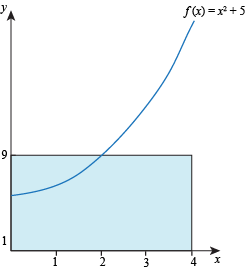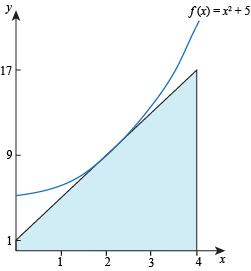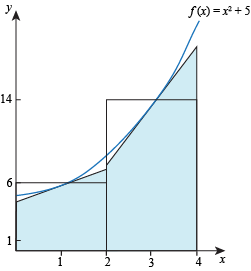Study Guide

# Midpoint Sum

## Midpoint Sum

We're driving along from right coast to the left coast, and now it's time to take a rest stop at the midpoint sum. Grab some snacks before continuing on. We recommend all flavors of Sun Chips and wasabi almonds.

A midpoint sum is similar to a left-hand sum or right-hand sum. For a midpoint sum, the height of the rectangle on a particular sub-interval is the value of f at the midpoint of that sub-interval. Hence the name midpoint sum.

As with left- and right-hand sums, we can also find a midpoint sum using graphs or tables—as long as there's enough information to find the midpoint of each sub-interval.

• ### Midpoint Sums with Shortcuts

Midpoint sum notation isn't as concise as left-hand sums and right-hand sum notation. However, we can still use similar shortcuts to find the values of f at all the points we need, add these values up, and then multiply by the width of a sub-interval. Check out the examples!

• ### Over or Under Estimates

To understand when the midpoint rule gives an underestimate and when it gives an overestimate, we need to draw some pictures.

Let R be the region between the function f(x) = x2 + 5 on the interval [0, 4]. Take a midpoint sum using only one sub-interval, so we only get one rectangle:The midpoint of our one sub-interval [0, 4] is 2. Draw the tangent line to the function f at x = 2. This tangent line "cuts off" a triangle from the rectangle. Now we have a new shape with the same area as the original rectangle.The new shape doesn't cover all of R. This means the area of the new shape is an underestimate for the area of R. Since the new shape and the original midpoint sum rectangle have the same area, the midpoint sum is also an underestimate for the area of R.

If we use more sub-intervals, the punchline is the same. The midpoint sum gives us one rectangle per sub-interval. We can replace each rectangle with a shape that has the same area and doesn't cover the entire area between the function and the x-axis on its sub-interval. For example, here are the original rectangles and new shapes if we use 2 sub-intervals:Now let S be the region between the function

f(x) = 17 – x2 and the x-axis on the interval [0, 4].

Take a midpoint sum using only one sub-interval. The midpoint of our one sub-interval [0, 4] is 2. Draw the tangent line to the function f at x = 2. Again, the tangent line cuts off part of the rectangle. We have a new shape with the same area as the original rectangle.

However, this time the new shape covers more area than it needs to. The area of the new shape is an overestimate for the area of S. Since the original rectangle has the same area as the new shape, the original midpoint sum was also an overestimate for the area of S.

To summarize: whether the midpoint sum provides an over- or -under- estimate depends on concavity. If the function is concave up, the tangent line lies under the graph so a midpoint sum produces an under-estimate. If the function is concave down, the tangent line lies over the graph so a midpoint sum produces an over-estimate. If the function has no concavity (is a straight line), a midpoint sum produces the exact area between the function and the x-axis on the specified interval. Whether the function is increasing or decreasing has no effect on whether a midpoint sum produces an underestimate or overestimate.